# Overview

This vignette aims to clarify the usage of the survtab_ag and survtab functions included in this package. survtab_ag estimates various survival functions and cumulative incidence functions (CIFs) non-parametrically using aggregated data, and survtab is a wrapper for survtab_ag, to which Lexis data is supplied.

Two methods (surv.method) are currently supported: The "lifetable" (actuarial) method only makes use of counts when estimating any of the supported survival time functions. The default method ("hazard"}) estimates appropriate hazards and transforms them into survival function or CIF estimates.

For relative survival estimation we need also to enumerate the expected hazard levels for the subjects in the data. This is done by merging expected hazards to individuals’ subintervals (which divide their survival time lines to a number of small intervals). For Pohar-Perme-weighted analyses one must additionally compute various weighted figures at the level of split subject data.

If one has subject-level data, the simplest way of computing survival function estimates with popEpi is by defining a Lexis object and using survtab, which will do the rest. For pre-aggregated data one may use the survtab_ag function instead. One can also use the lexpand function to split, merge population hazards, and aggregate in a single function call and then use survtab_ag if that is convenient.

# Using survtab

It is straightforward to estimate various survival time functions with survtab once a Lexis object has been defined (see ?Lexis in package Epi for details):

library(popEpi)
library(Epi)
data(sire)

## NOTE: recommended to use factor status variable
x <- Lexis(entry = list(FUT = 0, AGE = dg_age, CAL = get.yrs(dg_date)),
exit = list(CAL = get.yrs(ex_date)),
data = sire[sire$dg_date < sire$ex_date, ],
exit.status = factor(status, levels = 0:2,
labels = c("alive", "canD", "othD")),
merge = TRUE)
## NOTE: entry.status has been set to "alive" for all.
## pretend some are male
set.seed(1L)
x$sex <- rbinom(nrow(x), 1, 0.5) ## observed survival - explicit method st <- survtab(Surv(time = FUT, event = lex.Xst) ~ sex, data = x, surv.type = "surv.obs", breaks = list(FUT = seq(0, 5, 1/12))) ## observed survival - easy method (assumes lex.Xst in x is the status variable) st <- survtab(FUT ~ sex, data = x, surv.type = "surv.obs", breaks = list(FUT = seq(0, 5, 1/12))) ## printing gives the used settings and ## estimates at the middle and end of the estimated ## curves; more information available using summary() st ## ## Call: ## survtab(formula = FUT ~ sex, data = x, breaks = list(FUT = seq(0, 5, 1/12)), surv.type = "surv.obs") ## ## Type arguments: ## surv.type: surv.obs --- surv.method: hazard ## ## Confidence interval arguments: ## level: 95 % --- transformation: log-log ## ## Totals: ## person-time:23993 --- events: 3636 ## ## Stratified by: 'sex' ## sex Tstop surv.obs.lo surv.obs surv.obs.hi SE.surv.obs ## 1: 0 2.5 0.6174 0.6328 0.6478 0.007751 ## 2: 0 5.0 0.4962 0.5126 0.5288 0.008321 ## 3: 1 2.5 0.6235 0.6389 0.6539 0.007748 ## 4: 1 5.0 0.5006 0.5171 0.5334 0.008370 Plotting by strata (men = blue, women = red): plot(st, col = c("blue", "red"))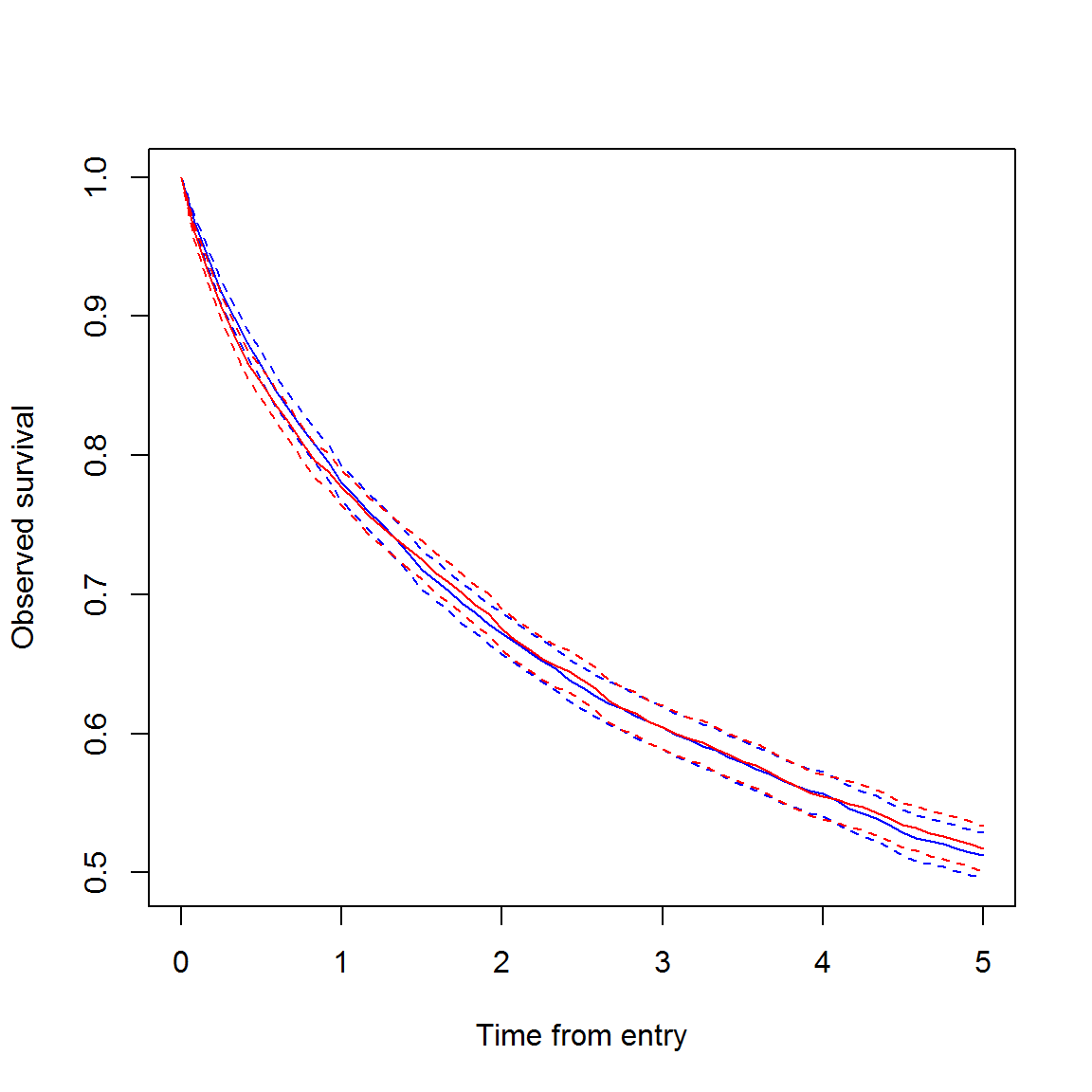Note that the correct usage of the formula argument in survtab specifies the time scale in the Lexis object over which survival is computed (here "FUT" for follow-up time). This is used to identify the appropriate time scale in the data. When only supplying the survival time scale as the right-hand-side of the formula, the column lex.Xst in the supplied Lexis object is assumed to be the (correctly formatted!) status variable. When using Surv() to be explicit, we effectively (and exceptionally) pass the starting times to the time argument in Surv(), and time2 is ignored entirely. The function will fail if time does not match exactly with a time scale in data. When using Surv(), one must also pass the status variable, which can be something other than the lex.Xst variable created by Lexis(), though usually lex.Xst is what you want to use (especially if the data has already been split using e.g. splitLexis or splitMulti, which is allowed). It is recommended to use a factor status variable to pass to Surv(), though a numeric variable will work in simple cases (0 = alive, 1 = dead; also FALSE = alive, TRUE = dead). Using Surv() also allows easy passing of transformations of lex.Xst, e.g. Surv(FUT, lex.Xst %in% 1:2). The argument breaks must be a named list of breaks by which to split the Lexis data (see ?splitMulti). It is mandatory to assign breaks at least to the survival time scale ("FUT" in our example) so that survtab knows what intervals to use to estimate the requested survival time function(s). The breaks also determine the window used: It is therefore easy to compute so called period estimates by defining the roof and floor along the calendar time scale, e.g. breaks = list(FUT = seq(0, 5, 1/12), CAL = c(2000, 2005)) would cause survtab to compute period estimates for 2000-2004 (breaks given here as fractional years, so 2005 is effectively 2004.99999…). ## Relative/net survival Relative/net survival estimation requires knowledge of the expected hazard levels for the individuals in the data. In survtab this is accomplished by passing a long-format data.frame of population hazards via the pophaz argument. E.g. the popmort dataset included in popEpi (Finnish overall mortality rates for men and women). data(popmort) pm <- data.frame(popmort) names(pm) <- c("sex", "CAL", "AGE", "haz") head(pm) ## sex CAL AGE haz ## 1 0 1951 0 0.036363176 ## 2 0 1951 1 0.003616547 ## 3 0 1951 2 0.002172384 ## 4 0 1951 3 0.001581249 ## 5 0 1951 4 0.001180690 ## 6 0 1951 5 0.001070595 The data.frame should contain a variable named "haz" indicating the population hazard at the level of one subject-year. Any other variables are considered to be variables, by which to merge population hazards to the (split) subject-level data within survtab. These merging variables may correspond to the time scales in the used Lexis object. This allows for e.g. merging in different population hazards for the same subject as they get older. The following causes survtab to estimate EdererII relative survival: st.e2 <- survtab(Surv(time = FUT, event = lex.Xst) ~ sex, data = x, surv.type = "surv.rel", relsurv.method = "e2", breaks = list(FUT = seq(0, 5, 1/12)), pophaz = pm) plot(st.e2, y = "r.e2", col = c("blue", "red"))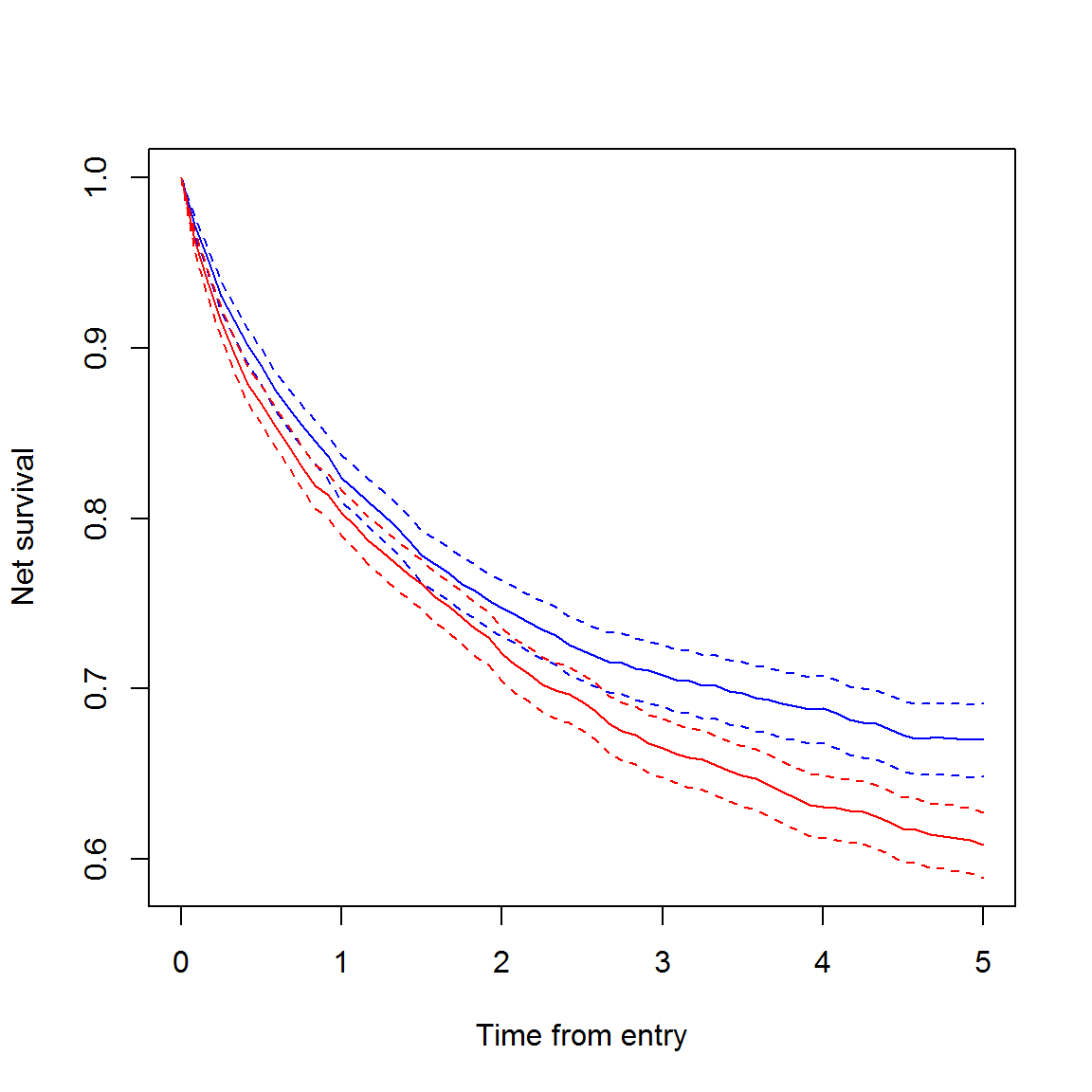Note that the curves diverge due to merging in the “wrong” population hazards for some individuals which we randomized earlier to be male though all the individuals in data are actually female. Pohar-Perme-weighted estimates can be computed by st.pp <- survtab(Surv(time = FUT, event = lex.Xst) ~ sex, data = x, surv.type = "surv.rel", relsurv.method = "pp", breaks = list(FUT = seq(0, 5, 1/12)), pophaz = pm) Compare with EdererII estimates: plot(st.e2, y = "r.e2", col = c("blue", "red"), lty = 1) lines(st.pp, y = "r.pp", col = c("blue", "red"), lty = 2)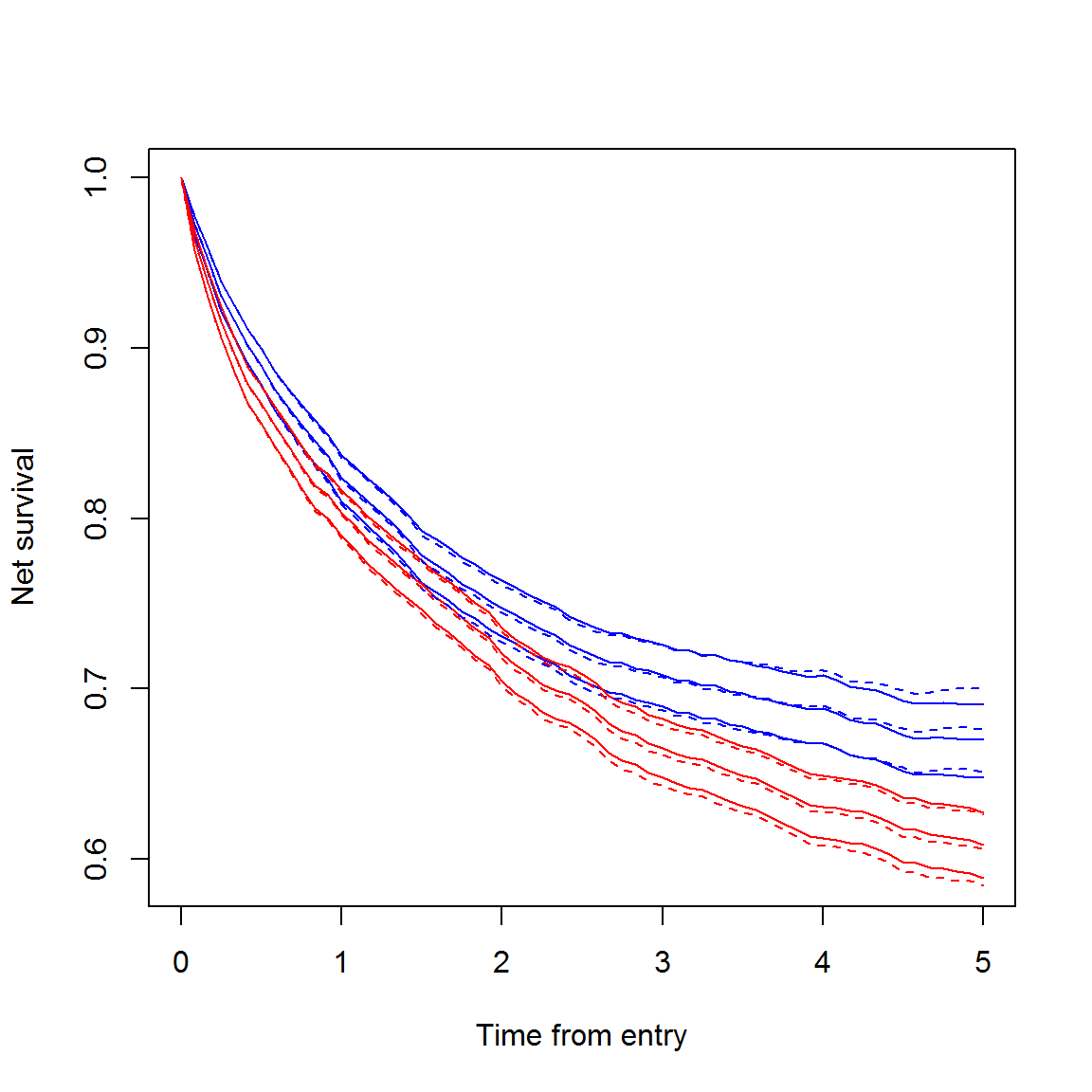## Adjusting estimates survtab also allows for adjusting the survival curves by categorical variables — typically by age groups. The following demonstrates how: ## an age group variable x$agegr <- cut(x$dg_age, c(0, 60, 70, 80, Inf), right = FALSE) ## using "internal weights" - see ?ICSS for international weights standards w <- table(x$agegr)
w
##
##   [0,60)  [60,70)  [70,80) [80,Inf)
##     1781     1889     2428     2129
w <- list(agegr = as.numeric(w))
st.as <- survtab(Surv(time = FUT, event = lex.Xst) ~ sex + adjust(agegr),
data = x, weights = w,
surv.type = "surv.rel", relsurv.method = "e2",
breaks = list(FUT = seq(0, 5, 1/12)),
pophaz = pm)
plot(st.as, y = "r.e2.as", col = c("blue", "red"))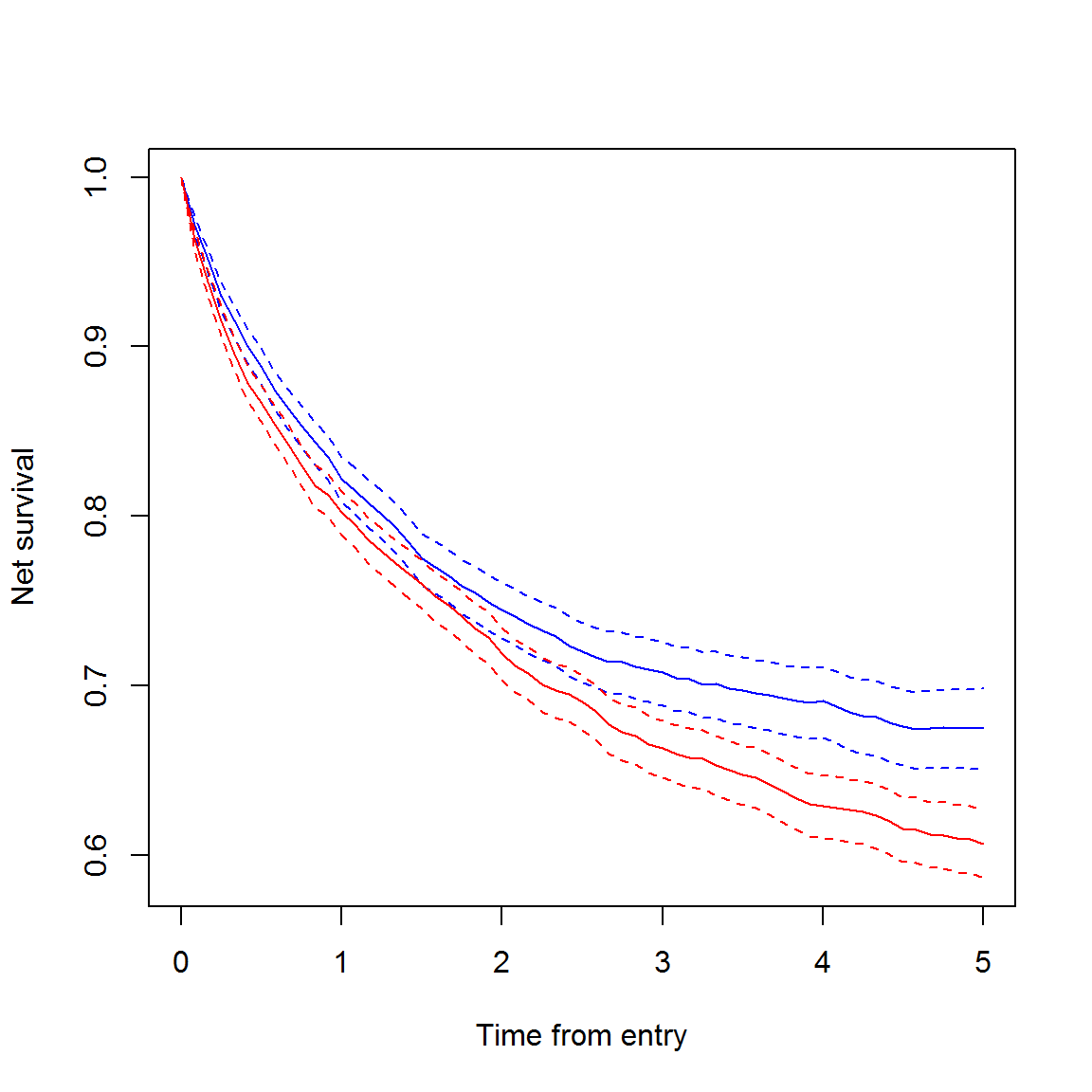We now have age-adjusted EdererII relative/net survival estimates. The weights argument allows for either a list of weights (with one or multiple variables to adjust by) or a data.frame of weights. Examples:

list(sex = c(0.4, 0.6), agegr = c(0.2, 0.2, 0.4, 0.2))
## $sex ##  0.4 0.6 ## ##$agegr
##  0.2 0.2 0.4 0.2
wdf <- merge(0:1, 1:4)
names(wdf) <- c("sex", "agegr")
wdf$weights <- c(0.1, 0.1, 0.1, 0.1, 0.2, 0.2, 0.1, 0.1) wdf ## sex agegr weights ## 1 0 1 0.1 ## 2 1 1 0.1 ## 3 0 2 0.1 ## 4 1 2 0.1 ## 5 0 3 0.2 ## 6 1 3 0.2 ## 7 0 4 0.1 ## 8 1 4 0.1 The weights do not have to sum to one when supplied as they are internally forced to do so within each stratum. In the data.frame of weights, the column of actual weights to use must be named “weights”. When there are more than one variable to adjust by, and a list of weights has been supplied, the variable-specific weights are first multiplied together (cumulatively) and then scaled to sum to one. This adjusting can be done to any survival time function that survtab (and survtab_ag) estimates. One can also supply adjusting variables via the adjust argument if convenient: st.as <- survtab(Surv(time = FUT, event = lex.Xst) ~ sex, adjust = "agegr", data = x, weights = w, surv.type = "surv.rel", relsurv.method = "e2", breaks = list(FUT = seq(0, 5, 1/12)), pophaz = pm) Where adjust could also be adjust = agegr, adjust = list(agegr) or adjust = list(agegr = cut(dg_age, c(0, 60, 70, 80, Inf), right = FALSE)) for exactly the same results. When adjusting by multiple variables, one must supply a vector of variable names in data or a list of multiple elements (as in the base function aggregate). ## Other survival time functions One can also estimate cause-specific survival functions, cumulative incidence functions (CIFs, a.k.a. crude risk a.k.a. absolute risk functions), and CIFs based on the excess numbers of events. Cause-specific survival is close to net survival as they are philosophically highly similar concepts: st.ca <- survtab(Surv(time = FUT, event = lex.Xst) ~ 1, data = x, surv.type = "surv.cause", breaks = list(FUT = seq(0, 5, 1/12))) st.pp <- survtab(Surv(time = FUT, event = lex.Xst) ~ 1, data = x, surv.type = "surv.rel", relsurv.method = "pp", breaks = list(FUT = seq(0, 5, 1/12)), pophaz = pm) plot(st.ca, y = "surv.obs.canD", col = "blue") lines(st.pp, y = "r.pp", col = "red")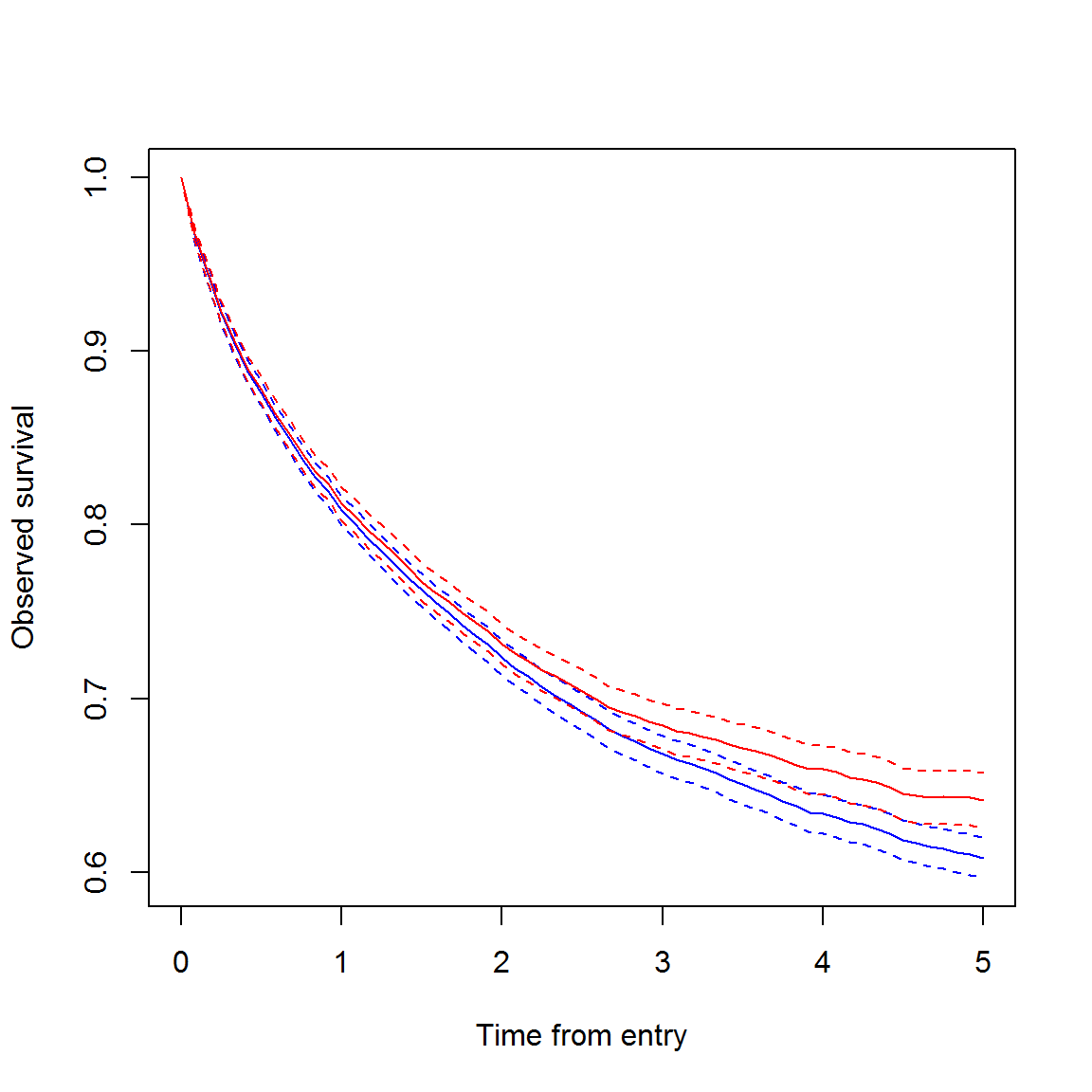Absolute risk: st.cif <- survtab(Surv(time = FUT, event = lex.Xst) ~ 1, data = x, surv.type = "cif.obs", breaks = list(FUT = seq(0, 5, 1/12))) plot(st.cif, y = "CIF_canD", conf.int = FALSE) lines(st.cif, y = "CIF_othD", conf.int = FALSE, col = "red")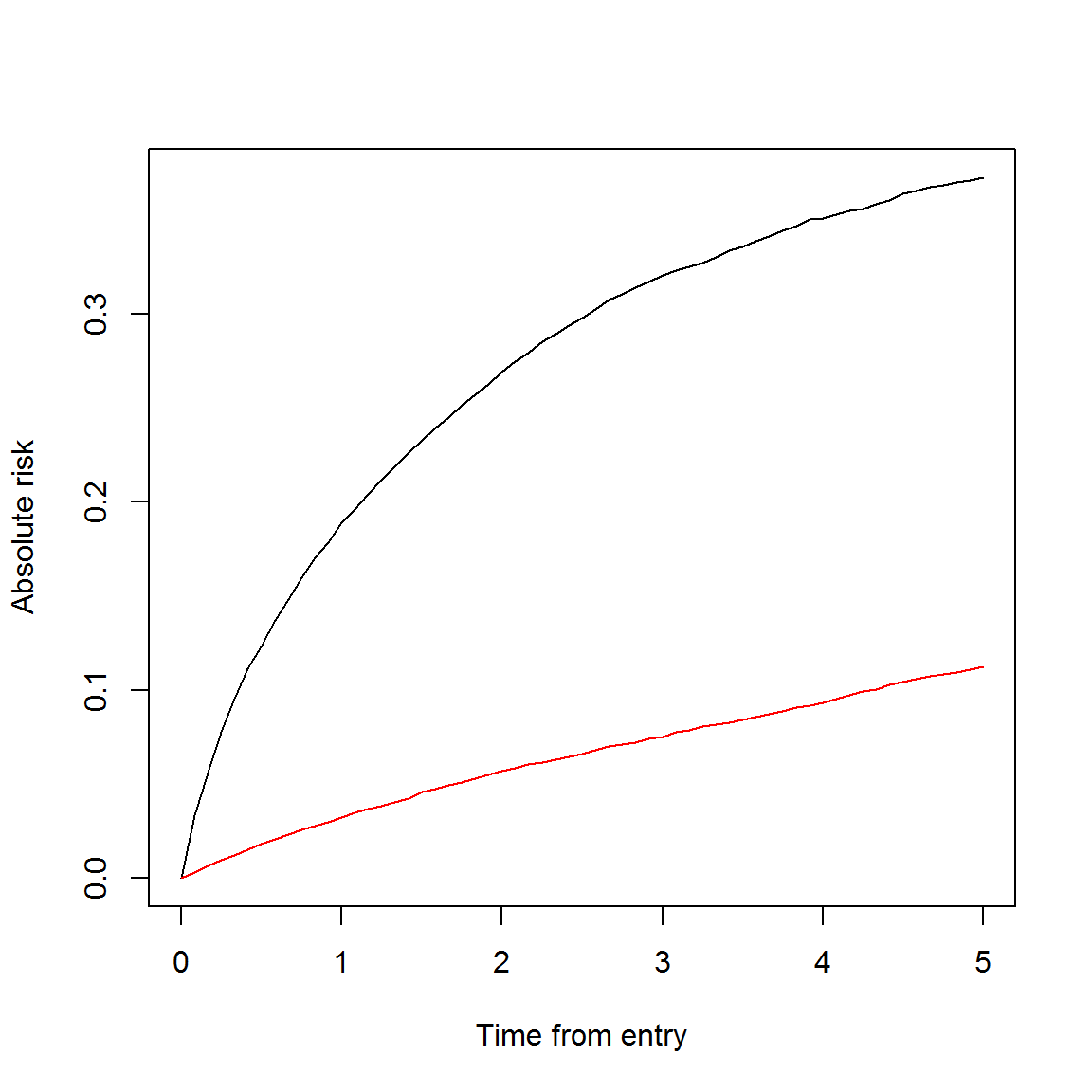The “relative CIF” attempts to be close to the true CIF without using knowledge about the types of events, e.g. causes of death: st.cir <- survtab(Surv(time = FUT, event = lex.Xst) ~ 1, data = x, surv.type = "cif.rel", breaks = list(FUT = seq(0, 5, 1/12)), pophaz = pm) plot(st.cif, y = "CIF_canD", conf.int = FALSE, col = "blue") lines(st.cir, y = "CIF.rel", conf.int = FALSE, col = "red")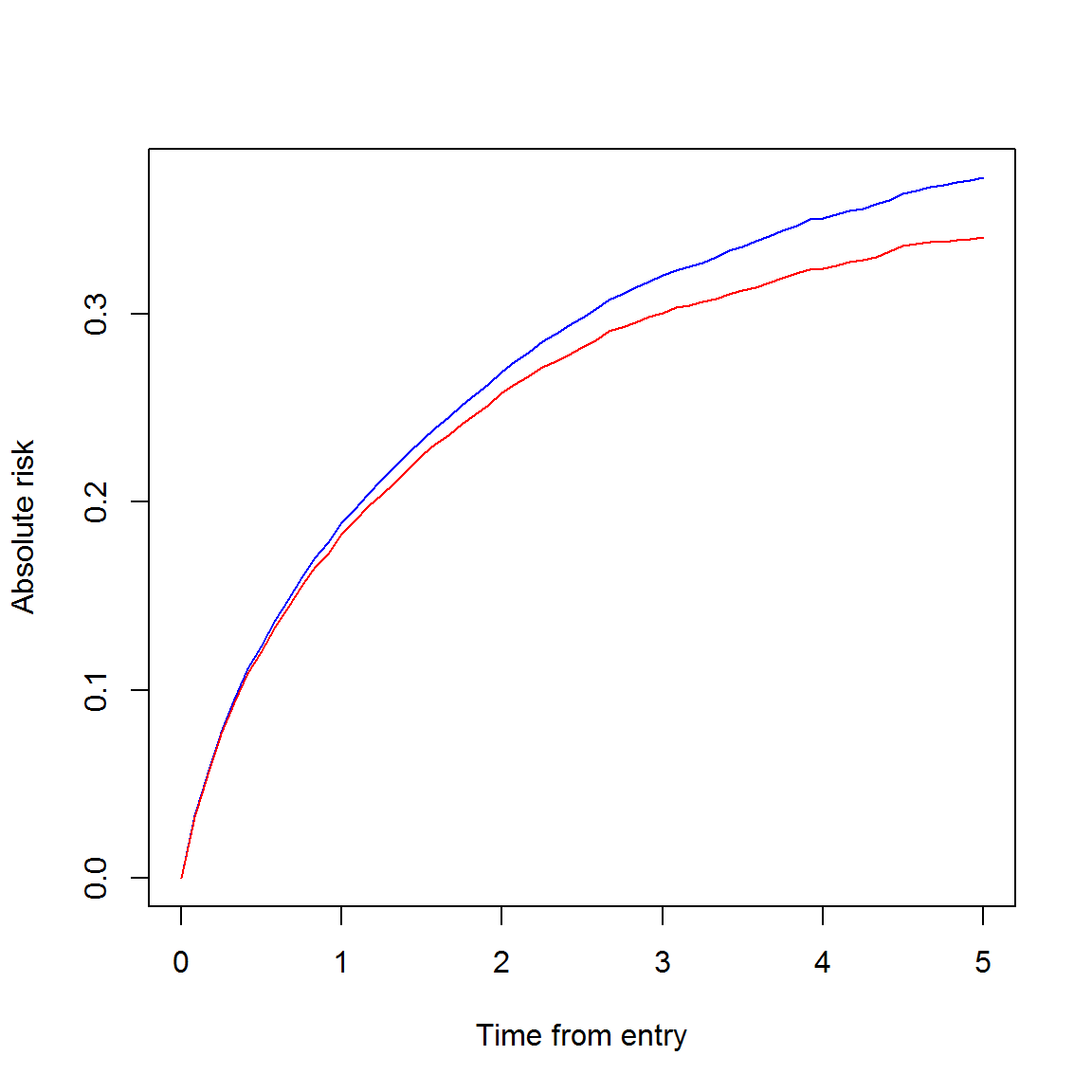# Using survtab_ag Arguments concerning the types and methods of estimating of survival time functions work the same in survtab_ag as in survtab (the latter uses the former). However, with aggregated data one must explicitly supply the various count and person-time variables. Also, usage of the formula argument is different. For demonstration purposes we form an aggregated data set using lexpand; see ?lexpand for more information on that function. sire$sex <- rbinom(nrow(sire), size = 1, prob = 0.5)
ag <- lexpand(sire, birth = "bi_date", entry = "dg_date", exit = "ex_date",
status = "status", breaks = list(fot = seq(0, 5, 1/12)),
aggre = list(sex, fot))
## dropped 16 rows where entry == exit
head(ag)
##    sex        fot     pyrs at.risk from0to0 from0to1 from0to2
## 1:   0 0.00000000 336.9447    4126       12      143       12
## 2:   0 0.08333333 323.8542    3959       19      101       17
## 3:   0 0.16666667 314.1913    3822       10       86       15
## 4:   0 0.25000000 305.0373    3711       15       77       13
## 5:   0 0.33333333 296.7300    3606       16       72       13
## 6:   0 0.41666667 289.3323    3505       13       50       14

Now simply do:

st <- survtab_ag(fot ~ sex, data = ag, surv.type = "surv.obs",
surv.method = "hazard",
d = c("from0to1", "from0to2"), pyrs = "pyrs")

Or:

st <- survtab_ag(fot ~ sex, data = ag, surv.type = "surv.obs",
surv.method = "lifetable",
d = c("from0to1", "from0to2"), n = "at.risk",
n.cens = "from0to0")

Note that e.g. argument d could also have been supplied as

list(from0to1, from0to2)

or

list(canD = from0to1, othD = from0to2)

for identical results. The last is convenient for e.g. surv.cause computations:

st.ca <- survtab_ag(fot ~ sex, data = ag, surv.type = "surv.cause",
surv.method = "hazard",
d = list(canD = from0to1, othD = from0to2), pyrs = "pyrs")
plot(st.ca, y = "surv.obs.canD", col = c("blue", "red"))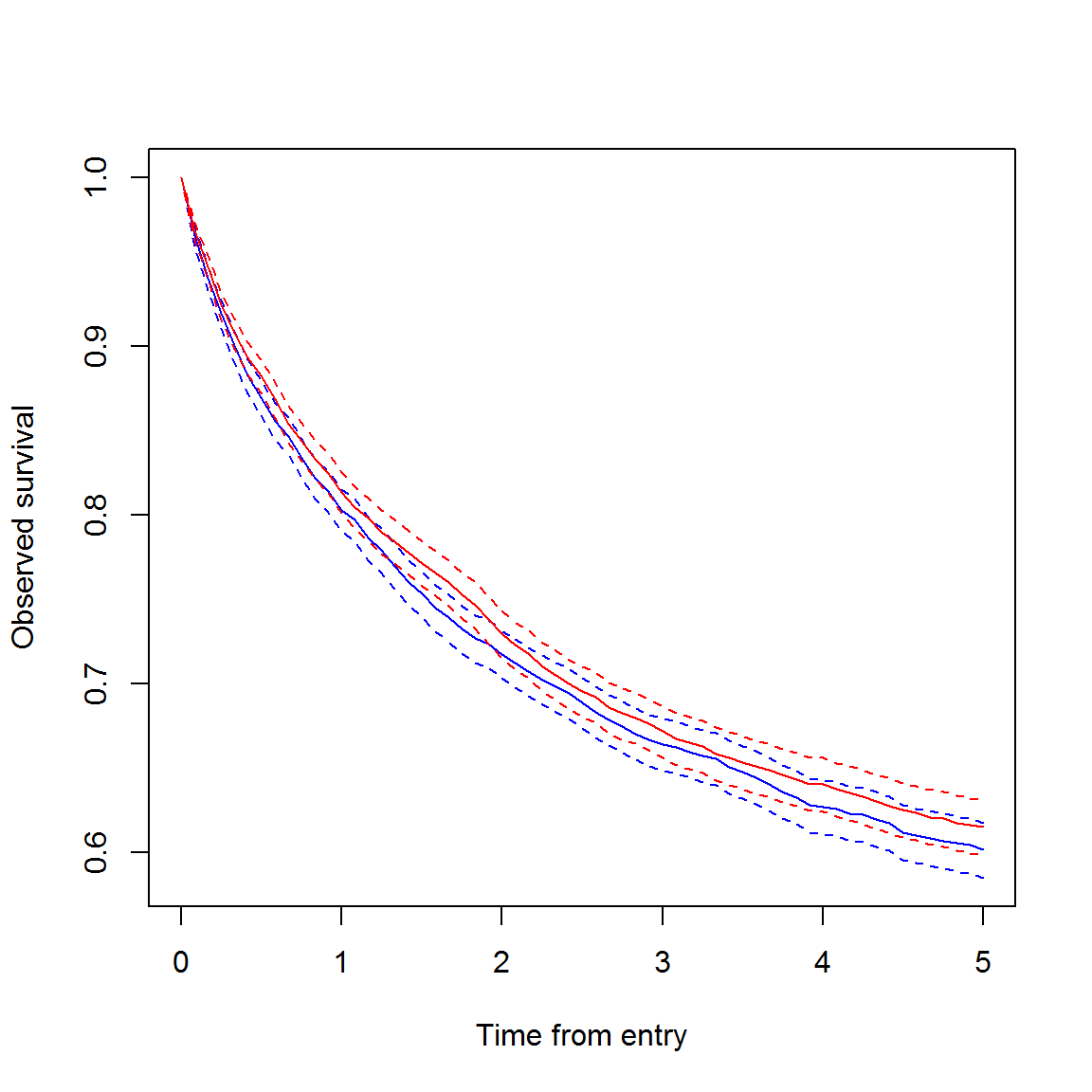One has to supply the most variables when computing Pohar-Perme estimates (though it is probably rare to have third-source aggregated data with Pohar-Perme weighted figures, it is implemented here to be used as a workhorse for survtab). For this we must aggregate again to get the Pohar-Perme weighted counts and subject-times:

ag <- lexpand(sire, birth = "bi_date", entry = "dg_date", exit = "ex_date",
status = "status", breaks = list(fot = seq(0, 5, 1/12)),
pophaz = popmort, pp = TRUE,
aggre = list(sex, fot))
## dropped 16 rows where entry == exit
## NOTE: 83 rows in split data had values of 'age' higher than max of pophaz's 'agegroup'; the hazard values at 'agegroup' == 100 were used for these
st.pp <- survtab_ag(fot ~ sex, data = ag, surv.type = "surv.rel",
surv.method = "hazard", relsurv.method = "pp",
d = list(from0to1 + from0to2), pyrs = "pyrs",
d.pp = list(from0to1.pp + from0to2.pp),
d.pp.2 = list(from0to1.pp.2 + from0to2.pp.2),
pyrs.pp = "ptime.pp", d.exp.pp = "d.exp.pp")
plot(st.pp, y = "r.pp", col = c("blue", "red"))`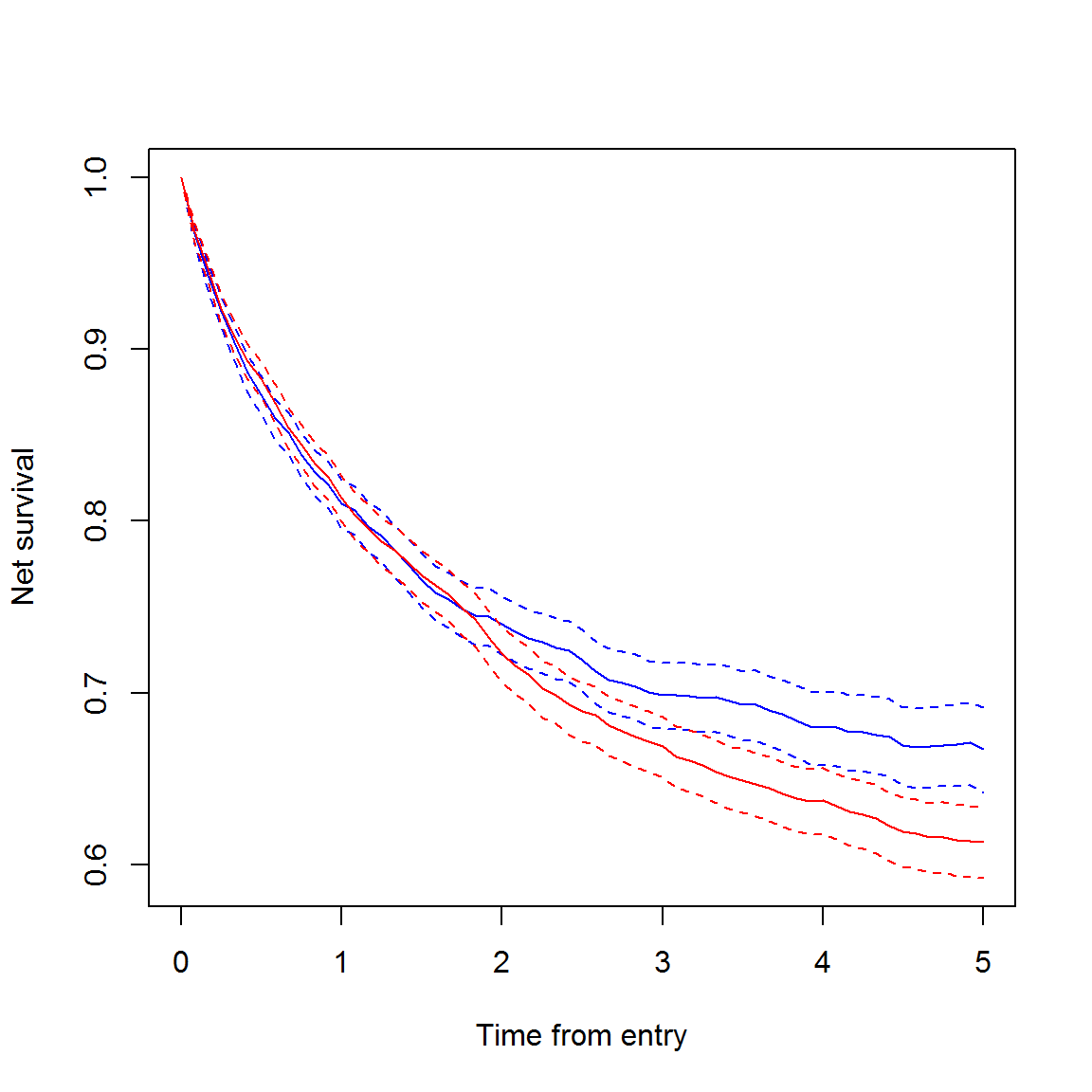Here it is best to supply only one column to each argument since Pohar-Perme estimates will not be computed for several types of events at the same time.